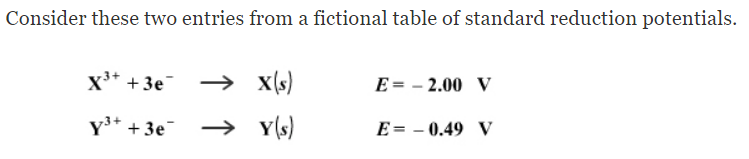# Problem: What is the standard potential of a cell where X is the anode and Y is the cathode?

###### FREE Expert Solution
80% (238 ratings)
###### Problem Details

What is the standard potential of a cell where X is the anode and Y is the cathode?What scientific concept do you need to know in order to solve this problem?

Our tutors have indicated that to solve this problem you will need to apply the Cell Potential concept. If you need more Cell Potential practice, you can also practice Cell Potential practice problems.

What is the difficulty of this problem?

Our tutors rated the difficulty ofWhat is the standard potential of a cell where X is the anod...as medium difficulty.

How long does this problem take to solve?

Our expert Chemistry tutor, Sabrina took 2 minutes and 37 seconds to solve this problem. You can follow their steps in the video explanation above.

What professor is this problem relevant for?

Based on our data, we think this problem is relevant for Professor Leslie's class at UCM.